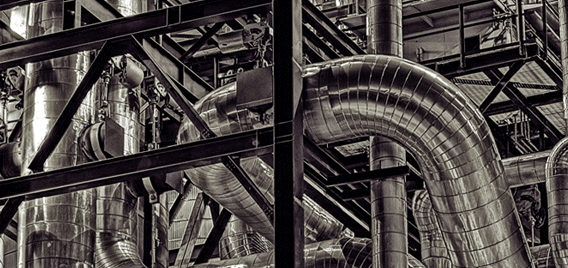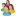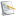### Chemical and Process Engineering Resources## Using Equivalent Lengths of Valves and Fittings

Nov 08 2010 01:20 PM | pleckner in Fluid FlowNomenclature

 D = Diameter, ft d = Diameter, inches f = Darcy friction factor ft = Darcy friction factor in the zone of complete turbulence g = Acceleration of gravity, ft/sec2 hL = Head loss in feet K = Resistance coefficient or velocity head loss K1 = K for the fitting at NRE = 1 K? = K value from CRANE L = Straight pipe length, ft Leq = Equivalent length of valve or fitting, ft NRE = Reynolds Number ?P = Pressure drop, psi n = Velocity, ft/sec W = Flow Rate, lb/hr ? = Density, lb/ft3

References

1. Crane Co., "Flow of Fluids through Valves, Fittings and Pipe", Crane Technical Paper No. 410, New York, 1991.
2. Hooper, W. B., The Two-K Method Predicts Head Losses in Pipe Fittings, Chem. Eng., p. 97-100, August 24, 1981.
3. Darby, R., Correlate Pressure Drops through Fittings, Chem. Eng., p. 101-104, July, 1999.
4. AIChE Center for Chemical Process Safety, "Guidelines for Pressure Relief and Effluent Handling systems", pp. 265-268, New York, 1998.

### Fluid Flow Articleslidachang

yeal,thank you very much,i got a lot from  this topic.ksb
In Crane Tech Paper 410 (1982 version), Example 4-14 shows a 5"x4" Reducing Elbow which they model as a straight size 5" elbow and a sudden enlargement 4" to 5". In step 4 of Example 4-14, they calculate a pressure increase (negative pressure drop) according to the Bernoulli theorem, because the water slows down as it flows from the smaller diameter to the larger diameter. I was wondering why you didn't include the velocity change in your Sulfuric Acid example since it flows from the 1" pump discharge nozzle through the reducer to the 3" pipe. Since the velocity in the 1" nozzle is 31.22 ft/s, the Bernoulli dP would be -11.72 psi plus the frictional dP of 7.24 psi for a total pressure drop of -4.48 psi which is a pressure increase.I'm a bit confused by the preferred equations to be used. The only equation that produces the 11.73 psi dP for the system described is Equation 2, yet Equations 6 & 7 seem to be preferred.

•Industrial Forum

•Student Forum

•Refining Forum

•Simulation Forum

•Relief Devices Forum

•Tank Venting Forum

•Separation Technology

•Calculations and Tips

•Fluid Flow

•Heat Transfer

•Maintenance and Repair

•Utilities

•Safety and Pressure Relief

•Bulk Solids

•Process and Reactions

•Energy

•Other Topics

•For Students

•Physical Properties

•Archived Articles